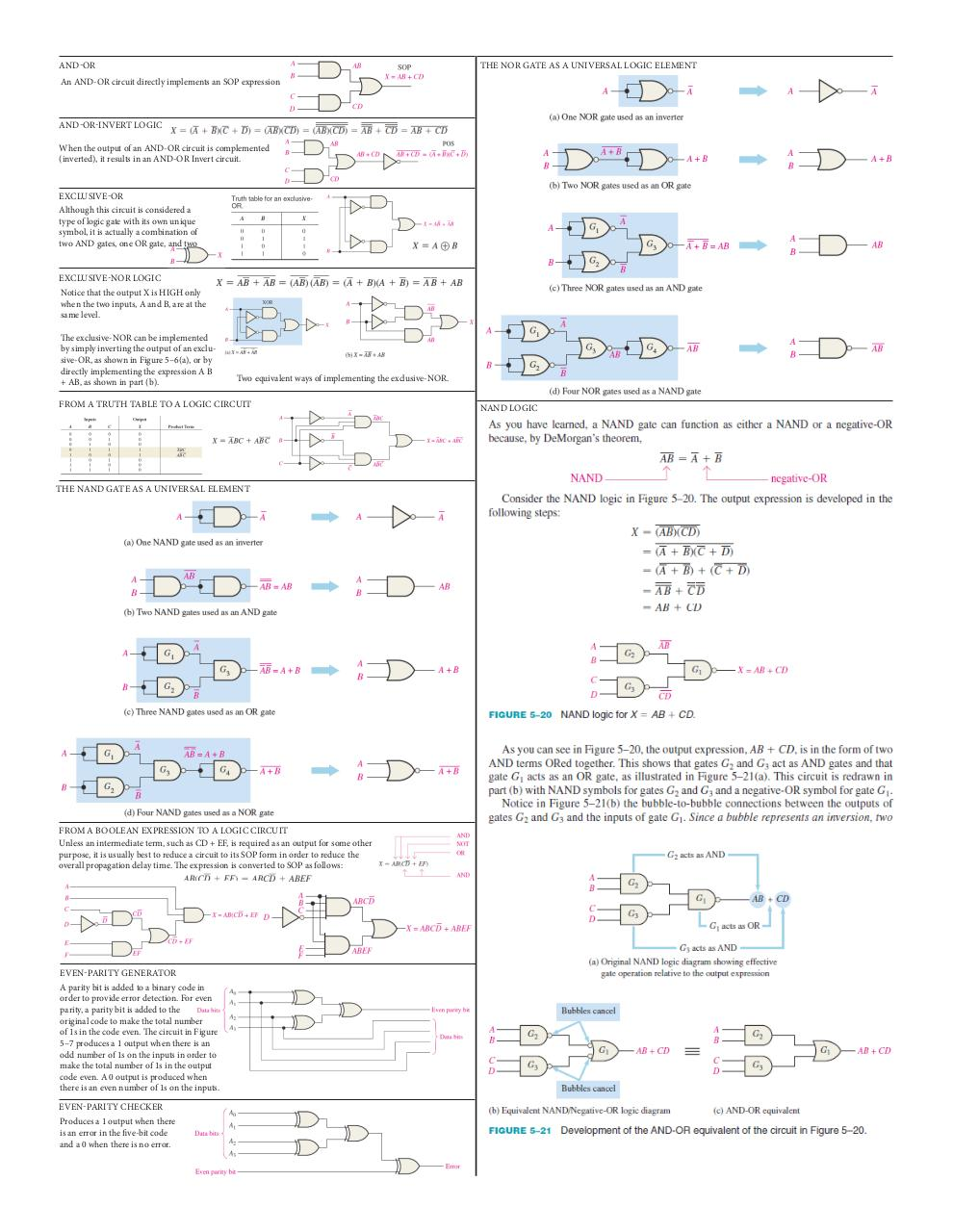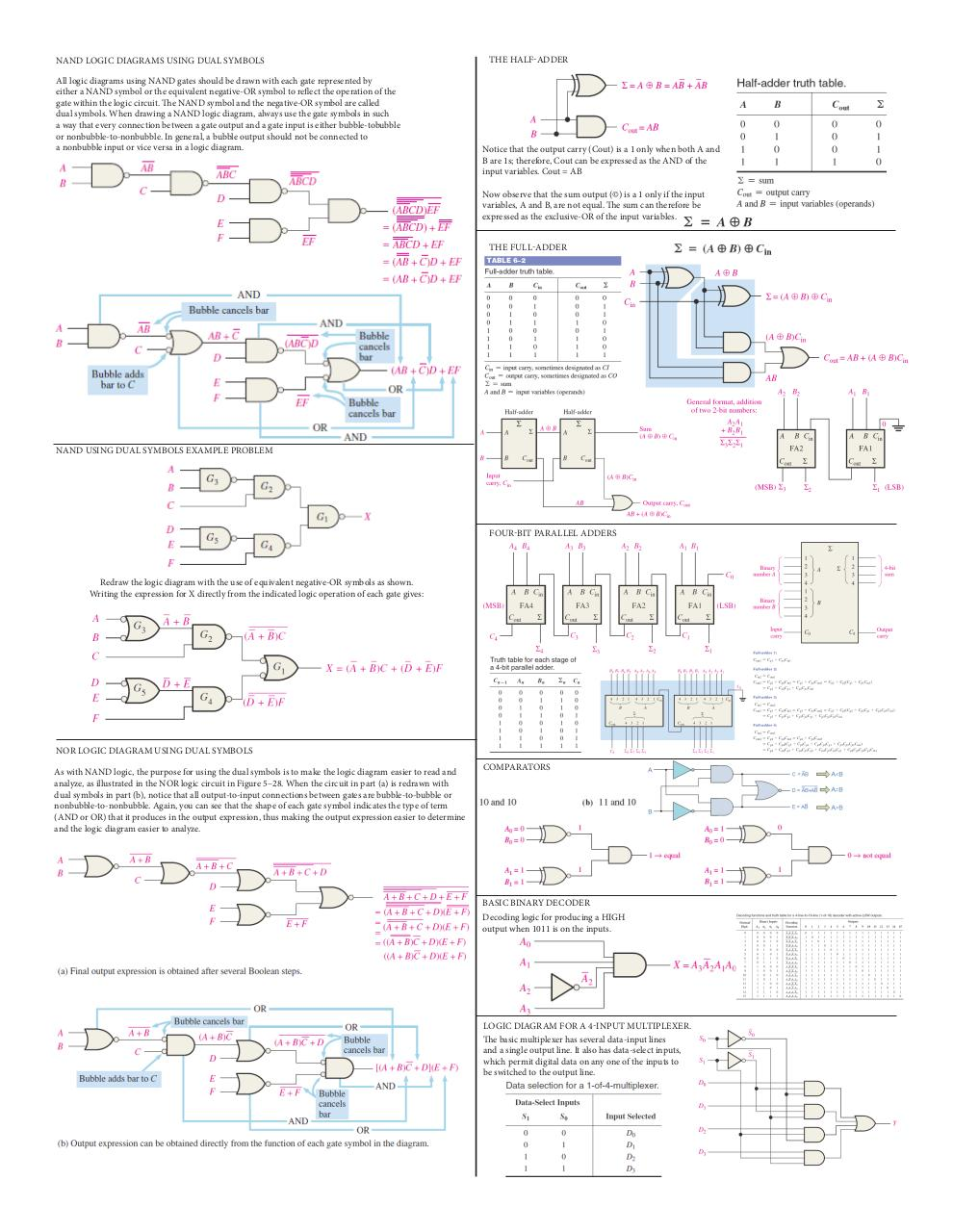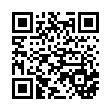# Cheat Sheet 3 .pdf

### File information

Original filename: Cheat Sheet 3.pdf

This PDF 1.4 document has been generated by Adobe InDesign CS6 (Windows) / Adobe PDF Library 10.0.1, and has been sent on pdf-archive.com on 20/10/2015 at 20:43, from IP address 73.1.x.x. The current document download page has been viewed 8700 times.
File size: 663 KB (2 pages).
Privacy: public file

Cheat Sheet 3.pdf (PDF, 663 KB)

### Document preview

AND-OR

THE NOR GATE AS A UNIVERSAL LOGIC ELEMENT

An AND-OR circuit directly implements an SOP expression

AND-OR-INVERT LOGIC
When the output of an AND-OR circuit is complemented
(inverted), it results in an AND-OR Invert circuit.

EXCLUSIVE-OR
Although this circuit is considered a
type of logic gate with its own unique
symbol, it is actually a combination of
two AND gates, one OR gate, and two
EXCLUSIVE-NOR LOGIC
Notice that the output X is HIGH only
when the two inputs, A and B, are at the
same level.
The exclusive-NOR can be implemented
by simply inverting the output of an exclusive-OR, as shown in Figure 5–6(a), or by
directly implementing the expression A B
+ AB, as shown in part (b).

Two equivalent ways of implementing the exclusive-NOR.

FROM A TRUTH TABLE TO A LOGIC CIRCUIT

THE NAND GATE AS A UNIVERSAL ELEMENT

FROM A BOOLEAN EXPRESSION TO A LOGIC CIRCUIT
Unless an intermediate term, such as CD + EF, is required as an output for some other
purpose, it is usually best to reduce a circuit to its SOP form in order to reduce the
overall propagation delay time. The expression is converted to SOP as follows:

EVEN-PARITY GENERATOR
A parity bit is added to a binary code in
order to provide error detection. For even
parity, a parity bit is added to the
original code to make the total number
of 1s in the code even. The circuit in Figure
5–7 produces a 1 output when there is an
odd number of 1s on the inputs in order to
make the total number of 1s in the output
code even. A 0 output is produced when
there is an even number of 1s on the inputs.

EVEN-PARITY CHECKER
Produces a 1 output when there
is an error in the five-bit code
and a 0 when there is no error.

NAND LOGIC

NAND LOGIC DIAGRAMS USING DUAL SYMBOLS
All logic diagrams using NAND gates should be drawn with each gate represented by
either a NAND symbol or the equivalent negative-OR symbol to reflect the operation of the
gate within the logic circuit. The NAND symbol and the negative-OR symbol are called
dual symbols. When drawing a NAND logic diagram, always use the gate symbols in such
a way that every connection between a gate output and a gate input is either bubble-tobubble
or nonbubble-to-nonbubble. In general, a bubble output should not be connected to
a nonbubble input or vice versa in a logic diagram.

Notice that the output carry (Cout) is a 1 only when both A and
B are 1s; therefore, Cout can be expressed as the AND of the
input variables. Cout = AB
Now observe that the sum output (©) is a 1 only if the input
variables, A and B, are not equal. The sum can therefore be
expressed as the exclusive-OR of the input variables.

NAND USING DUAL SYMBOLS EXAMPLE PROBLEM

Redraw the logic diagram with the use of equivalent negative-OR symbols as shown.
Writing the expression for X directly from the indicated logic operation of each gate gives:

NOR LOGIC DIAGRAM USING DUAL SYMBOLS
As with NAND logic, the purpose for using the dual symbols is to make the logic diagram easier to read and
analyze, as illustrated in the NOR logic circuit in Figure 5–28. When the circuit in part (a) is redrawn with
dual symbols in part (b), notice that all output-to-input connections between gates are bubble-to-bubble or
nonbubble-to-nonbubble. Again, you can see that the shape of each gate symbol indicates the type of term
(AND or OR) that it produces in the output expression, thus making the output expression easier to determine
and the logic diagram easier to analyze.

COMPARATORS

BASIC BINARY DECODER
Decoding logic for producing a HIGH
output when 1011 is on the inputs.

LOGIC DIAGRAM FOR A 4-INPUT MULTIPLEXER.
The basic multiplexer has several data-input lines
and a single output line. It also has data-select inputs,
which permit digital data on any one of the inputs to
be switched to the output line.#### HTML Code

Copy the following HTML code to share your document on a Website or Blog

#### QR Code### Related keywords